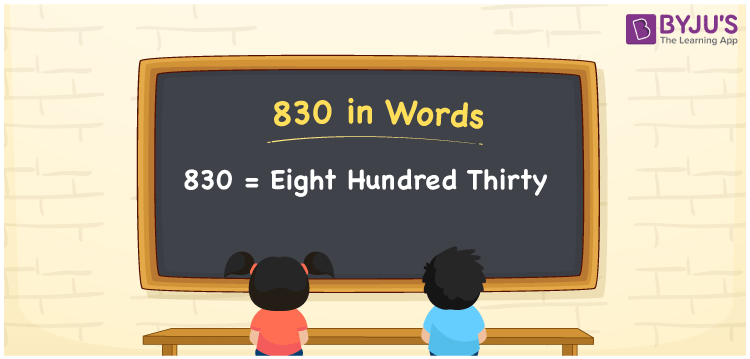# 830 in Words

830 in words is written as eight hundred thirty. The name of number 830 in English is “eight hundred thirty”. The word eight hundred thirty is used in many real-life situations. For example, there are eight hundred thirty students in a classroom. Thus, it is a counting number. Also, to represent the currency equal to 830, we can write it in words as Rupees eight hundred thirty or eight hundred thirty rupees. Therefore, it is necessary to learn the numbers in words, for the ease of understanding and expressing them.

 830 in words Eight hundred thirty eight hundred thirty in Numbers 830

## 830 in English Words## How to Write 830 in Words?

If we know the place value of digits of 830, then we can easily express it in words. The place value is basically the position of a digit in a number. 830 is a three-digit number, therefore, we can specify the position of each digit of 830 in a place value chart. In the Indian numbering system, the order of place value of digits from right to left is given by:

 Hundreds Tens Ones 8 3 0

We can write it in expanded form as:

8 × Hundred + 3 × Ten + 0 × One

= 8 × 100 + 3 × 10 + 0 × 1

= 800 + 30

= 830

= eight hundred thirty

Therefore, 830 in words is written as eight hundred thirties.

830 is a natural number that precedes 831 and succeeds 829.

830 in words – eight hundred thirty

Is 830 an odd number? – No

Is 830 an even number? – Yes

Is 830 a perfect square number? – No

Is 830 a perfect cube number? – No

Is 830 a prime number? – No

Is 830 a composite number? – Yes

## Frequently Asked Questions on 830 in Words

### How to write 830 in words?

830 in English is written as “eight hundred thirty”.

### Is 830 an odd number or even number?

830 is an even number because it is wholly divisible by 2.

### Write eight hundred thirty in numbers.

eight hundred thirty in numbers is 830.